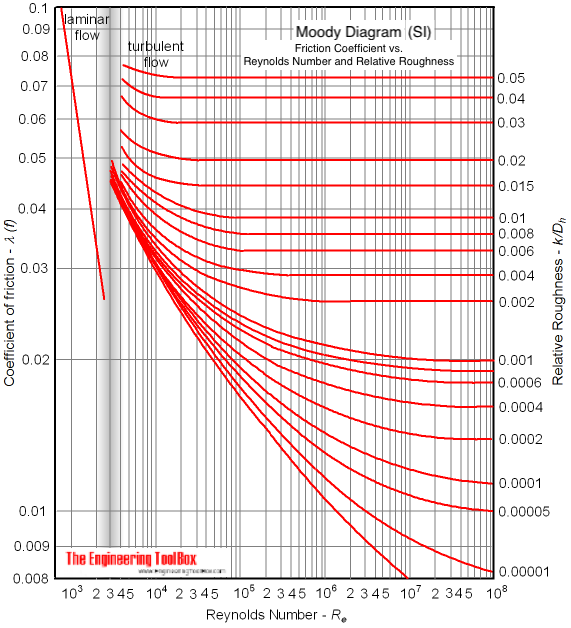Engineering ToolBox - Resources, Tools and Basic Information for Engineering and Design of Technical Applications!

# Moody Diagram

## Calculate fluid flow friction coefficients from a Moody diagram.

There are two definitions of the friction factor

• SI based
• Imperial based

The SI based friction factor is four times larger than the Imperial based friction factor.

### SI based Moody Diagram

The Moody friction factor - λ (or f) - is used in the Darcy-Weisbach major loss equation. The coefficient can be estimated with the diagram below:If the flow is transient - 2300 < Re < 4000 - the flow varies between laminar and turbulent flow and the friction coefiicient is not possible to determine. The friction factor can usually be interpolated between the laminar value at Re = 2300 and the turbulent value at Re = 4000.

### Example - SI based Friction Factor

For a PVC pipe with absolute roughness  k = 0.0015 10-3 (m), hydraulic diameter dh = 0.01 (m) and Reynolds number Re = 107 - the relative rougness can be calculated as

r = k / dh

= (0.0015 10-3 m) / (0.01 m)

= 0.00015

From the diagram above, with the relative rougness and the Reynolds number - the friction factor can be estimated to aprox. 0.013

### Alternative Moody Diagram

A very nice Moody diagram in pdf-format from Glasgow College of Nautical Studies can be found here:## Related Topics

• ### Fluid Mechanics

The study of fluids - liquids and gases. Involving velocity, pressure, density and temperature as functions of space and time.

## Related Documents

• ### Colebrook Equation

Friction loss coefficients in pipes, tubes and ducts.
• ### Energy Equation - Pressure Loss vs. Head Loss

Calculate pressure loss - or head loss - in ducts, pipes or tubes.
• ### Reynolds Number

Introduction and definition of the dimensionless Reynolds Number - online calculators.
• ### Surface Roughness vs. Mechanical Processing

Mechanical production methods and their surface roughness.

## Engineering ToolBox - SketchUp Extension - Online 3D modeling!

Add standard and customized parametric components - like flange beams, lumbers, piping, stairs and more - to your Sketchup model with the Engineering ToolBox - SketchUp Extension - enabled for use with older versions of the amazing SketchUp Make and the newer "up to date" SketchUp Pro . Add the Engineering ToolBox extension to your SketchUp Make/Pro from the Extension Warehouse !

We don't collect information from our users. More about

## Citation

• The Engineering ToolBox (2003). Moody Diagram. [online] Available at: https://www.engineeringtoolbox.com/moody-diagram-d_618.html [Accessed Day Month Year].

Modify the access date according your visit.

9.29.12